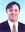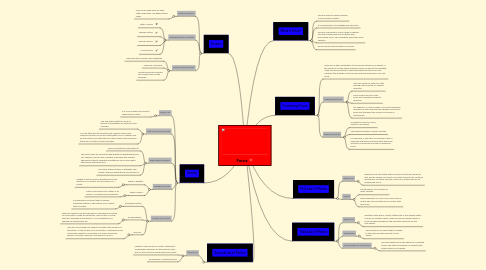# ForcePatrick Carlos-De Clercq
Get Started. It's FreeForce## 2. Combining Forces

### 2.2. Unbalanced Forces

2.2.1. This can cause an object to start moving, stop moving, or change direction.

2.2.2. They usually result in a net force and change the objects direction.

2.2.3. For example, if a boy pushes a box in the opposite direction of a girl the boy has a greater amount of force and therefore the forces on the box is unbalanced.

### 2.3. Balanced Forces

2.3.1. An exqual amount of force acting on an object.

2.3.2. The objects direction doesn't change.

2.3.3. For example, if two girls are pushing a box in opposite directions and they exert the same amount of force this is known as balanced force.

## 3. Friction

### 3.1. What is Friction?

3.1.1. Two forces that exert on each other when they rub against each other.

### 3.2. Different types of Friction

3.2.1. Static Friction

3.2.2. Sliding Friction

3.2.3. Rolling Friction

3.2.4. Fluid Friction

### 3.3. What causes Friction?

3.3.1. How hard two surfaces push together.

3.3.2. The type of surface.

3.3.3. Smooth surfaces produce less friction then rough surfaces.

## 4. Gravity

### 4.1. What is it?

4.1.1. It is a force that pulls objects towards each other.

### 4.2. Law of Universal Gravity

4.2.1. This law states that the force of gravity acts between all objects in the universe.

4.2.2. You are attracted to the Earth and objects around you. Objects around you are also attracted to you. However you do not notice your attraction to other objects because your attraction to Earth is much stronger.

### 4.3. What affects gravity?

4.3.1. Mass and distance affect gravity.

4.3.2. The more mass an object has the greater its gravitational pull. For example, the sun has a greater mass than the planets therefore it exerts a greater gravitational force and makes the planets orbit around it.

4.3.3. The more distance there is between two objects the less gravitational force there is.

### 4.4. Weight and Mass

4.4.1. What is weight?

4.4.1.1. Weight is the amount of gravitational force exerted on an object on the surface of a planet.

4.4.2. What is mass?

4.4.2.1. Mass is the amount of matter in an object. It is measured in kilograms.

### 4.5. Gravity and Motion

4.5.1. Projectile motion

4.5.1.1. A projectile is an object that is thrown. Projectile motion is the motion of an object that is thrown.

4.5.2. Air Resistance

4.5.2.1. When an objects falls through the air it experiences a type of fluid friction called air resistance. This friction in in the direction opposite to the object. It is an upward force exerted on objects that fall.

4.5.3. Free Fall

4.5.3.1. Free fall occurs when an object is falling to the surface of the Earth. In free fall the force of gravity is unbalanced and causes the object to accelerate. For every second an object is falling its velocity increases by 9.8 m/s.

## 5. First Law of Motion

### 5.1. What is it?

5.1.1. Newtons first law states that an object at rest will remain at rest, and an object moving at a constant velocity will continue moving at a constant velocity, unless it is acted upon by an unbalanced force.

### 5.2. Inertia

5.2.1. The tendency of an object to resist change.

5.2.2. Inertia depends on mass. The more mass an object has more inertia than an object with less mass.

## 6. Second Law of Motion

### 6.1. What is it?

6.1.1. Newtons second law of motion states that acceleration depends on the object's mass and on the net force acting upon the object.

6.1.2. Acceleration= Netforce/Mass

## 7. Third Law of Motion

### 7.1. What is it?

7.1.1. Newtons third law of motion states that if one object exerts a force on another object, then the second object exerts a force of equal strength in the opposite direction on the first object.

### 7.2. Momentum

7.2.1. The moving of an object that is related to the mass and the velocity of the object

### 7.3. Conservation of Momentum

7.3.1. This law states that in the absence of outside forces, the total momentum of objects that interact does not change.